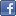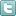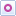EE Times-India > Memory/Storage

Memory/Storage# Power tip: How to power DDR memory

Posted: 25 Nov 2011Print Version

Keywords:DDR  memory clocks  sink current

Power dissipation within CMOS logic systems is mainly related to clock frequency, input capacitance of the different gates within the system, and the supply voltage. As device feature sizes and, hence, supply voltages have been reduced, significant gains have been made in lowering dissipation at the gate level.

These reduced dissipations and higher-speed operation of the lower-voltage devices have allowed system clock frequencies to be pushed up into the gigahertz range. At these very-high clock frequencies, controlled impedances, properly terminated busses and minimal cross-coupling provide a high-fidelity clock signal.

Traditionally, logic systems have been designed to clock data on only one edge of the clock, while the double data rate (DDR) memory clocks on both the leading and falling edge of the clock. This doubles the data through-put while slightly increasing system power dissipation.

The increased data rate requires that the clock-distribution network be carefully designed to minimise ringing and reflections, which may inadvertently clock-logic devices. Two candidate bus-termination schemes are presented in the figure.Figure: VTT termination voltages reduce termination power by half.

In the first schematic (A), bus-termination resistors are placed at the end of the distribution network and are connected to ground. If the bus driver is in the low state, the resistors have zero dissipation. In the high state, the resistors dissipate power equal to the supply voltage (VDD) squared, divided by the bus resistance (source impedance plus termination resistor). The average loss is the supply-voltage squared divided by twice the bus resistance.

In the second scheme (B), the termination resistor is connected to a supply voltage (VTT) that is half the VDD voltage. The dissipation in the resistor is then constant, regardless of the supply voltage and equal to VTT (or (Vdd/2)) squared divided by the termination resistance. This results in a factor-of two-power savings when compared with the first approach, but at the cost of needing an additional power supply.

Note that the requirements on this power supply are somewhat unique:

• First, its output needs be half that of the driver voltage (VDD).
• Second, it needs to both source and sink current. When the driver output voltage is low, current flows from the VTT supply. However, when the driver is high, current flows into the supply.
• Third, the supply needs to transition between modes as the system data changes and must provide a low-source impedance, up to near the clock rate of the system.
Peak power is relatively easy to determine from the termination resistors, the clock frequency, and the capacitances within the system. Average power is harder to estimate and can be many times less than one-tenth of the peak power. You need to consider such things as the fact that the system is dynamic and does not really have a fixed clocking rate, data is not clocked every cycle, and there will be devices that are tri-stated.

Average current is an important number to verify with system measurements as it can be important for determining the appropriate power supply topology. For example, you would trade the low-power dissipation of a switching power supply against the low cost and size of a linear regulator.

 Related Articles Editor's Choice
Comment on "Power tip: How to power DDR memory"

Top Ranked Articles

WebinarsVisit Asia Webinars to learn about the latest in technology and get practical design tips.

Search EE Times India
Services

Go to topConnect on FacebookFollow us on TwitterFollow us on Orkut
﻿